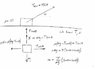# Tension and friction

• leroyjenkens

## Homework Statement

An initially stationary box of sand is to be pulled across a floor by means of a cable in which the tension should not exceed 792 N. The coefficient of static friction between the box and the floor is 0.37. (a) What should be the angle between the cable and the horizontal in order to pull the greatest possible amount of sand, and (b) what is the weight of the sand and box in that situation?

## Homework Equations

Maybe F=ma? I have no idea where to begin on this. So little information is given it makes me think there's some mistake.

## The Attempt at a Solution

I drew a box with a string attached to it and that's all I could figure out. The book gives no examples that are even within a light year of being similar to this, so that thing is worthless.

You want to maximize m(θ) = C(sinθ + cosθ)

see,

#### Attachments

•sand082.jpg
21 KB · Views: 359
You want to maximize m(θ) = C(sinθ + cosθ)

see,

Thanks for the response.

I still don't quite see how I can figure out the problem. I don't have enough numerical values to plug in, from what I can tell.

Plot sinθ + cosθ

You want to maximize m(θ) = C(sinθ + cosθ)

Set d m(θ)/dθ = 0